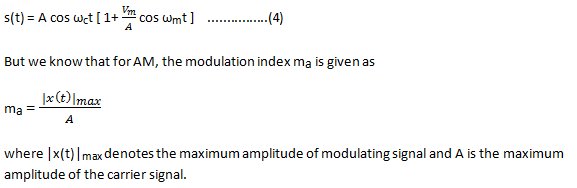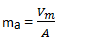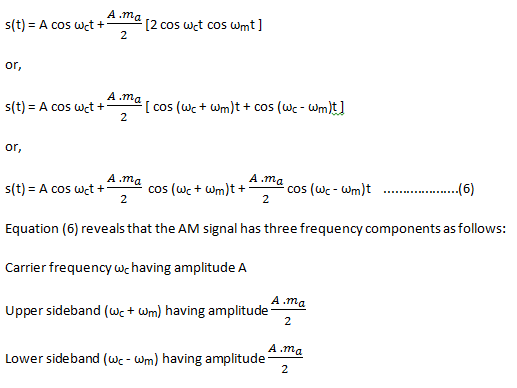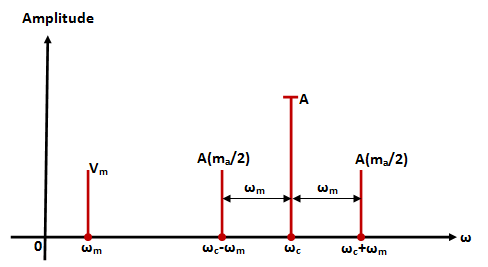# Derive an expression for Single Tone Amplitude Modulated Wave and draw its frequency spectrum

## Single Tone Amplitude Modulation (AM) Definition

Till now, we discussed amplitude modulation in which we assumed that baseband or modulating signal is a random signal which contains a large number of frequency components. This means that a carrier signal (fixed frequency signal) is modulated by a large number of frequency components.

In this section we shall discuss amplitude modulation in which the modulating or baseband signal consists of only one (single) frequency i.e. modulation is done by a single frequency or tone. This type of amplitude modulation is known as single tone amplitude modulation .

## Mathematical Expression

Let us consider a single tone modulating signal as :

x(t) = Vm cos ωmt         ……………….(1)

which contains a single frequency ωm.

Signal x(t) may be a voltage signal or a current signal. Here, we have assumed that x(t) is a voltage signal with maximum amplitude equal to Vm.

Let the carrier signal be :

c(t) = A cos ωct         ……………….(2)

We know that the general expression for AM signal is :

s(t) = [A + x(t)] cos ωct

or,

s(t) = A cos ωct + x(t) cos ωct       ………………..(3)

Putting the value of x(t), we get

s(t) = A cos ωct + Vm cos ωmt cos ωct

or,

s(t) = A cos ωct + Vm cos ωct  cos ωmt

or,In this case, we have

|x(t)|max  = Vm

Therefore,Putting this value ma of in equation (4) we get

s(t) = A cos ωct [ 1+ ma cos ωmt ]    …………….(5)

This is the desired expression for single-tone modulated signal.

The expression in equation (4) may further be simplified to observe the frequency components present in AM signal.

s(t) = A cos ωct [ 1+ ma cos ωmt ]

or,

s(t) = A cos ωct + A . ma cos ωct cos ωmt

or,## Frequency Spectrum

With the help  of these frequency components, we can plot the frequency spectrum of single-tone amplitude modulated (AM) wave . Figure 1 shows the one-sided frequency spectrum of single-toned AM wave.Fig 1: Single-sided frequency spectrum of single-tone AM wave